# Search Results (38)

View
Selected filters:
• Look At Physics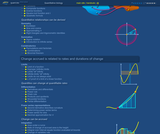Conditions of Use:
Remix and Share
Rating

In this video, we explore some concepts fundamental to algebra. To streamline the discussion of relationships between physical quantities, we introduce variables, functions, composition, and inverse. By thinking about the concept of an inverse function, we obtain our first glimpse of the imaginary root (i.e. square-root of -1) and the complex plane.

Subject:
Mathematics
Algebra
Material Type:
Lecture Notes
Provider:
Look At Physics
Provider Set:
A Mathematical Way to Think About Biology
Author:
David Liao
10/08/2011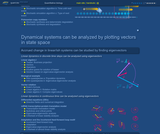Conditions of Use:
Remix and Share
Rating

In the master-equation formalism, a set of differential equations describe the time-evolution of the probability distribution of an ensemble of systems. This can be used, for example, to describe the varied mRNA copy numbers found in individual cells in a population.

Subject:
Life Science
Mathematics
Chemistry
Physics
Material Type:
Lecture Notes
Provider:
Look At Physics
Provider Set:
A Mathematical Way to Think About Biology
Author:
David Liao
10/08/2012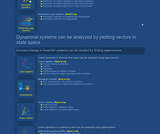Conditions of Use:
Remix and Share
Rating

The stochastic simulation algorithm (SSA, Kinetic Monte Carlo, Gillespie algorithm) produces an example trajectory for a particular member of a probabilistic ensemble by looping over the following steps. The current state of the system is used to determine the likelihood of each possible chemical reaction in relative comparison to the likelihoods for the other possible reactions, as well as to determine when the next reaction is expected. Pseudo-random numbers are drawn to "roll the dice" to determine exactly when the next reaction will proceed, and which kind of reaction it will happen to be.

Subject:
Life Science
Mathematics
Chemistry
Physics
Material Type:
Lecture Notes
Provider:
Look At Physics
Provider Set:
A Mathematical Way to Think About Biology
Author:
David Liao
10/08/2012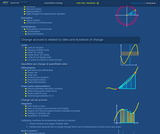Conditions of Use:
Remix and Share
Rating

In the first part of this video introduction to combinatorics, we introduce factorial notation to efficiently describe the ways we can arrange distinguishable objects in a row. Next, we count the combinations of L objects that can be grabbed from a container of L + N total objects. Finally, we use the formula for combinations to derive the binomial theorem.

Subject:
Mathematics
Material Type:
Lecture Notes
Provider:
Look At Physics
Provider Set:
A Mathematical Way to Think About Biology
Author:
David Liao
10/08/2012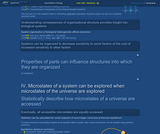Conditions of Use:
Remix and Share
Rating

In this deterministic cellular automata model, interactions between neighboring automata are described using a prisoner's dilemma. Limiting dispersal of seeds of annual plants can permit heterogeneous co-existence, whereas thorough mixing instead allows one subpopulation to dominate quickly.

Subject:
Life Science
Ecology
Forestry and Agriculture
Mathematics
Material Type:
Lecture Notes
Provider:
Look At Physics
Provider Set:
A Mathematical Way to Think About Biology
Author:
David Liao
10/08/2012Conditions of Use:
Remix and Share
Rating

In this video, we use direction fields (drawn as quiver plots) to illustrate the numerical integration of differential equations. We include a heuristic example of how one might try to adapt step size by comparing different orders of approximation.

Subject:
Mathematics
Material Type:
Lecture Notes
Provider:
Look At Physics
Provider Set:
A Mathematical Way to Think About Biology
Author:
David Liao
10/08/2012Conditions of Use:
Remix and Share
Rating

In the first video, we present a biological circuit topology from Ma, Trusina, El-Samad, Lim, and Tang, "Defining network topologies that can achieve biochemical adaptation," Cell, 138: 760-773 (2009). This topology supports adaptation, which is not the absence of change in response to stimulation/stress, but, instead, the ability to produce delayed compensation for those changes. In the second video, we summarize the method of almost linear stability analysis used to solve for the dynamics of this example system.

Subject:
Life Science
Mathematics
Material Type:
Lecture Notes
Provider:
Look At Physics
Provider Set:
A Mathematical Way to Think About Biology
Author:
David Liao
10/08/2012Conditions of Use:
Remix and Share
Rating

To describe how oscillations are supported in systems of differential equations, we present a classic "Romeo and Juliet" picture of two-dimensional oscillations, and we analyze how trajectories change as nullclines are arranged at different angles in the phase plane. In addition to models based on traditional systems of differential equations, dynamical systems with time delays and dynamical systems with stochastic fluctuation (i.e. stochastic resonance) can also support oscillations.

Subject:
Life Science
Mathematics
Material Type:
Lecture Notes
Provider:
Look At Physics
Provider Set:
A Mathematical Way to Think About Biology
Author:
David Liao
10/08/2012Conditions of Use:
Remix and Share
Rating

In the five parts of this video, we define the derivative and then build a cribsheet of rules for expressing the slopes of simple functions and combinations of functions. These include the power rule, the chain rule, the product and quotient rules, and the rules for differentiating sinusoidal functions.

Subject:
Mathematics
Material Type:
Lecture Notes
Provider:
Look At Physics
Provider Set:
A Mathematical Way to Think About Biology
Author:
David Liao
10/08/2012Conditions of Use:
Remix and Share
Rating

The first video segment presents a canonical mathematical example from quantitative biology, in which mRNA is transcribed from a gene sequence, and protein is translated from mRNA. The second segment uses eigenvector-eigenvalue analysis to sketch the trajectories of the system in a phase portrait. Finally, the third segment generalizes the linear stability analysis used to study this example.

Subject:
Life Science
Mathematics
Material Type:
Lecture Notes
Provider:
Look At Physics
Provider Set:
A Mathematical Way to Think About Biology
Author:
David Liao
10/08/2012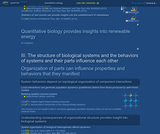Conditions of Use:
Remix and Share
Rating

Biological systems (e.g. cells) can make stochastic transitions between phenotypes (e.g. states of relatively increased or decreased drug resistance). This means that an initially drug-sensitive population can generate relatively drug-resistant subpopulations. This video presents a metronomogram, which is a tool for understanding whether such stochastic transitions can provide an opportunity for therapeutic treatment. Citation: Liao D, Estevez-Salmeron L, and Tlsty TD (2012) "Conceptualizing a tool to optimize therapy based on dynamic heterogeneity," Phys. Biol. 9:065005.

Subject:
Life Science
Mathematics
Physics
Material Type:
Lecture Notes
Provider:
Look At Physics
Provider Set:
A Mathematical Way to Think About Biology
Author:
David Liao
10/08/2012Conditions of Use:
Remix and Share
Rating

We express the exponential function of an imaginary variable in terms of sine and cosine. The "complex exponentials" that result trace out a circle in the complex plane. Pointing to one of the positions in the complex plane, we obtain the identity exp(i pi) = -1.

Subject:
Mathematics
Material Type:
Lecture Notes
Provider:
Look At Physics
Provider Set:
A Mathematical Way to Think About Biology
Author:
David Liao
10/08/2012Conditions of Use:
Remix and Share
Rating

In the first video segment, we introduce Euler's number by considering the problem of interest compounded continuously. After we obtain the power-series representation for exp(x), we explore its properties, in the next four video segments, to convince ourselves that exp(x) is literally an exponential function, meaning a number, approximately 2.71828, taken to the power x. In the final two segments, we present the natural logarithm and demonstrate that it is the anti-derivative of 1/x.

Subject:
Mathematics
Material Type:
Lecture Notes
Provider:
Look At Physics
Provider Set:
A Mathematical Way to Think About Biology
Author:
David Liao
10/08/2011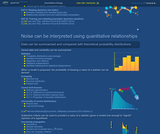Conditions of Use:
Remix and Share
Rating

In the first video segment, we analyze the population dynamics for a test-tube of cells that affect each others' likelihoods of replication when they collide. The particular example we use is a prisoner's dilemma, which has the almost paradoxical property that survival of the relatively most fit leads overall fitness to decrease. In the second video segment, we suggest that the population dynamics from the first segment can be related to an analysis that uses payoff matrices found in traditional game theory.

Subject:
Life Science
Ecology
Forestry and Agriculture
Mathematics
Material Type:
Lecture Notes
Provider:
Look At Physics
Provider Set:
A Mathematical Way to Think About Biology
Author:
David Liao
10/08/2012Conditions of Use:
Remix and Share
Rating

In the first part of this video, we review the idea of flat space and the Pythagorean theorem. The second part of the video reviews ideas from trigonometry including the unit circle, sine, cosine, and pi. We conclude with a proof of an angle-sum identity.

Subject:
Mathematics
Geometry
Material Type:
Lecture Notes
Provider:
Look At Physics
Provider Set:
A Mathematical Way to Think About Biology
Author:
David Liao
10/08/2011Conditions of Use:
Remix and Share
Rating

In the first video segment, we present a cartoon model of a weighted chain, which can be regarded as an approximation for a polymer under tension (e.g. a strand of DNA being stretched out using optical tweezers). The Hamiltonian and partition function for this system are described in the second segment. Finally, in the third segment, we calculate the average energy and elongation of the chain.

Subject:
Life Science
Mathematics
Chemistry
Physics
Material Type:
Lecture Notes
Provider:
Look At Physics
Provider Set:
A Mathematical Way to Think About Biology
Author:
David Liao
10/08/2012Conditions of Use:
Remix and Share
Rating

In this video, we become familiar with integrals, both by understanding them as sums of areas under plots of functions, and by understanding them as anti-derivatives. We present the u-substitution rule, which is the analog of the chain rule for differentiating composite functions.

Subject:
Mathematics
Material Type:
Lecture Notes
Provider:
Look At Physics
Provider Set:
A Mathematical Way to Think About Biology
Author:
David Liao
10/08/2011Conditions of Use:
Remix and Share
Rating

Students will see how the idea of a limit can be presented both in formal epsilon-delta-style terms, and using corresponding animations. After calculating a limit for a simple example function, we point out that limits do not always exist.

Subject:
Mathematics
Material Type:
Lecture Notes
Provider:
Look At Physics
Provider Set:
A Mathematical Way to Think About Biology
Author:
David Liao
10/08/2012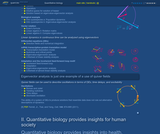Conditions of Use:
Remix and Share
Rating

The first video segment presents a "freemium" business model as a motivating example. The second and third segments provide mathematical background on vectors, vector spaces, operators, and representations. Using this theoretical foundation, we solve for the dynamics of the example business using eigenvalue-eigenvector analysis.

Subject:
Mathematics
Algebra
Material Type:
Lecture Notes
Provider:
Look At Physics
Provider Set:
A Mathematical Way to Think About Biology
Author:
David Liao
10/08/2012Conditions of Use:
Remix and Share
Rating

Using the complex exponentials described in the previous video, we show how oscillating systems can be modeled using "rotation matrices." One strategy for determining whether a dynamical system supports oscillations is to look for complex eigenvectors and eigenvalues.

Subject:
Mathematics
Algebra
Material Type:
Lecture Notes
Provider:
Look At Physics
Provider Set:
A Mathematical Way to Think About Biology
Author:
David Liao